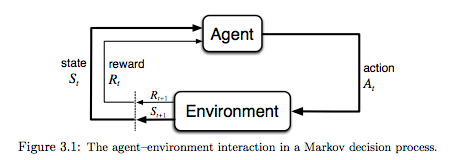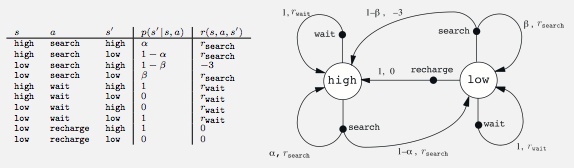In :
# %load /Users/facai/Study/book_notes/preconfig.py
%matplotlib inline

import matplotlib.pyplot as plt
import seaborn as sns
sns.set(color_codes=True)
#sns.set(font='SimHei', font_scale=2.5)
#plt.rcParams['axes.grid'] = False

import numpy as np

import pandas as pd
#pd.options.display.max_rows = 20

#import sklearn

#import itertools

#import logging
#logger = logging.getLogger()

#from IPython.display import SVG
from IPython.display import Image


# Chapter 3: Finite Markov Decision Processes ¶

MDP(Markov Decision Processes): actions influence not just immediate rewards, but also subsequential situations.

### 3.1 The Agent-Environment Interface¶

In :
Image('./res/fig3_1.png')

Out:finite MDP: the sets of states, actions and rewards all have a finite number of elements.

\begin{align} & p(s', r \mid s, a) \doteq \operatorname{Pr} \{ S_t = s', R_t = r \mid S_{t-1} = s, A_{t-1} = a \} \\ & \displaystyle \sum_{s' \in \mathcal{S}} \sum_{r \in \mathcal{R}} p(s', r \mid s, a) = 1 \quad \text{, for all $s \in \mathcal{S}$, $a \in \mathcal{A}(s)$} \end{align}
• state-transition probabilities:
\begin{align*} p(s' \mid s, a) & \doteq \operatorname{Pr} \{ S_t = s' \mid S_{t-1} = s, A_{t-1} = a \} \\ & = \sum_{r \in \mathcal{R}} p(s', r \mid s, a) \end{align*}
• expected rewards for state-action paris:
\begin{align*} r(s, a) & \doteq \mathbb{E} \left [ R_t \mid S_{t-1} = s, A_{t-1} = a \right ] \\ & = \sum_{r \in \mathcal{R}} \left ( r \sum_{s' \in \mathcal{S}} p(s', r \mid s, a) \right ) \end{align*}
• expected rewards for state-action-next-state triples:
\begin{align*} r(s, a, s') & \doteq \mathbb{E} \left [ R_t \mid S_{t-1} = s, A_{t-1} = a, S_t = s' \right ] \\ & = \sum_{r \in \mathcal{R}} r \frac{p(s', r \mid s, a)}{p(s' \mid s, a)} \end{align*}

agent-environment boundary represents the limit of the agent's absolute control, not of its knowledge.

In :
# Transition Graph
Image('./res/ex3_3.png')

Out:##### Exercise 3.4¶

$r(S_t, a) \; \pi(a \mid S_t)$

### 3.2 Goals and Rewards¶

goal: to maximize the total amount of reward it receives.

In particular, the reward signal is not the place to impart to the agent prior knowledge about how to achieve what it to do.

### 3.3 Returns and Episodes¶

• episodic tasks: $G_t \doteq R_{t+1} + R_{t+2} + R_{t+3} + \cdots + R_T$, $\quad G_t$ is expected return.
• continuing tasks: $G_t \doteq R_{t+1} + \gamma R_{t+2} + \gamma^2 R_{t+3} + \cdots = \displaystyle \sum_{k=0}^\infty \gamma^k R_{t+k+1} = R_{t+1} + \gamma G_{t+1}$
• $\gamma$ is called discount rate, and $0 \leq \gamma \leq 1$
• $G_T = 0$ often makes it easy to compute returns from reward sequences.
##### exercise 3.8¶

0 for escaping from the maze and -1 at all other times

##### exercise 3.10¶

$G_1 = \frac{7}{1 - r} = 70$

$G_0 = R_1 + 0.9 G_1 = 65$

##### exercise 3.11¶
\begin{align} G_t &= \sum_{k=0}^\infty r^k \\ &= 1 + r \sum_{k=0}^\infty r^k \\ &= 1 + r G_t \\ &= \frac1{1 - r} \end{align}

### 3.4 Unified Notation for Episodic and Continuing Tasks¶

$G_t \doteq \sum_{k=t+1}^T \gamma^{k-t-1} R_k$, including the possibility that $T = \infty$ or $\gamma = 1$.

### 3.5 Policies and Value Functions¶

• value functions: estimate how good it is for the agent to be in a given state.
• policy $\pi$: a mapping from states to probabilities of selecting each possible action.
• The value of a state $s$ under a policy $\pi$, denoted $v_\pi(s)$, is the expected return when starting in $s$ and following $\pi$ thereafter:
$v_\pi(s) \doteq \mathbb{E}_\pi [ G_t \mid S_t = s ]$
• $v_\pi$: state-value function for policy $\pi$
• $q_\pi$: action-value function for policy $\pi$, $q_\pi(s, a) \doteq \mathbb{E}_\pi [ G_t \mid S_t = s, A_t = a]$

Bellman equation for $v_\pi$:

\begin{equation} v_\pi(s) \doteq \displaystyle \sum_a \pi(a \mid s) \sum_{s', r} p(s', r \mid s, a) \left [ r + \gamma v_\pi(s') \right ] \quad \text{, for all $s \in \mathcal{S}$} \end{equation}

The value functions $v_\pi$ and $q_\pi$ can be estimated from experience, say Monte Carlo methods (average).

In :
# Example 3.5
from scipy.signal import convolve2d

reward_matrix = np.zeros((5, 5))
# kernel
kernel = np.array([[0, 1, 0],
[1, 0, 1],
[0, 1, 0]])

iteration_nums = 100

for _ in range(iteration_nums):
reward = convolve2d(reward_matrix, kernel, mode='same', boundary='fill', fillvalue=-1)
reward /= 4.0
# A -> A'
reward[0, 1] = 10 + reward[-1, 1]
# B -> B'
reward[0, -2] = 5 + reward[2, -2]
reward_matrix = reward

pd.DataFrame(reward_matrix)

Out:
0 1 2 3 4
0 2.253150 9.592586 4.524364 6.244556 1.271214
1 1.420013 3.970976 3.260312 2.897431 0.840299
2 0.455929 1.610993 1.648482 1.244556 0.192553
3 -0.207290 0.368589 0.478065 0.239763 -0.314645
4 -0.653676 -0.407414 -0.344568 -0.448925 -0.690892
##### exercise 3.12¶

(2.3 + 0.4 - 0.4 + 0.7) / 4 = 0.75

##### exercise 3.14¶

$\sum_{k=0}^\infty \gamma^k C = \frac{C}{1 - r}$ = constant offset

### 3.6 Optimal Policies and Optimal Value Functions¶

optimal policy:

\begin{align} v_\ast(s) & \doteq \displaystyle \max_\pi v_\pi(s) \quad \text{ for all } s \in \mathcal{S} \\ & = \max_a \sum_{s', r} p(s', r \mid s, a) \left [ r + \gamma v_\ast(s') \right ] \\ \end{align}

Any policy that is greedy with respect to the optimal evaluation function $v_\ast$ is an optimal policy.

optimal action-value function:

\begin{align} q_\ast(s, a) & \doteq \max_\pi q_\pi(s, a) \quad \text{ for all $s \in \mathcal{S}$ and $a \in \mathcal{A}(s)$} \\ & = \mathbb{E} [ R_{t+1} + \gamma v_\ast(S_{t+1}) \mid S_t = s, A_t = a ] \\ & = \sum_{s', r} p(s', r \mid s, a) \left [ r + \gamma \max_{a'} q_\ast (s', a') \right ] \\ \end{align}

Explicitly solving the Bellman optimality equation relies on at least three assumptions that are rarely true in practice:

1. we accurately know the dynamics of the environment;
2. we have enough computational resources;
3. the Markov property.

### 3.7 Optimality and Approximation¶

extreme computational cost, memory => approximations

put more effort into learning to make good decisions for frequently encountered states, at the expense of less effort for infrequently encountered states.

In [ ]: